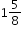# MA.6.NSO.3.5Export Print
Rewrite positive rational numbers in different but equivalent forms including fractions, terminating decimals and percentages.

### Examples

The numbercan be written equivalently as 1.625 or 162.5%

### Clarifications

Clarification 1: Rational numbers include decimal equivalence up to the thousandths place.
General Information
Subject Area: Mathematics (B.E.S.T.)
Strand: Number Sense and Operations
Status: State Board Approved

## Related Courses

This benchmark is part of these courses.
1205010: M/J Grade 6 Mathematics (Specifically in versions: 2014 - 2015, 2015 - 2022, 2022 and beyond (current))
1205020: M/J Accelerated Mathematics Grade 6 (Specifically in versions: 2014 - 2015, 2015 - 2020, 2020 - 2022, 2022 and beyond (current))
1204000: M/J Foundational Skills in Mathematics 6-8 (Specifically in versions: 2014 - 2015, 2015 - 2022, 2022 and beyond (current))
7812015: Access M/J Grade 6 Mathematics (Specifically in versions: 2014 - 2015, 2015 - 2018, 2018 - 2022, 2022 and beyond (current))

## Related Access Points

Alternate version of this benchmark for students with significant cognitive disabilities.
MA.6.NSO.3.AP.5: Rewrite a positive rational number 3 or less, as a fraction, decimal or a percent.

## Related Resources

Vetted resources educators can use to teach the concepts and skills in this benchmark.

## Educational Game

Fraction Quiz:

Test your fraction skills by answering questions on this site. This quiz asks you to simplify fractions, convert fractions to decimals and percentages, and answer algebra questions involving fractions. You can even choose difficulty level, question types, and time limit.

Type: Educational Game

## Lesson Plan

Predicting the decimal equivalent for a fraction - terminating or repeating?:

This lesson encourages students to make an important discovery. Will a given fraction yield a terminating or repeating decimal? Discussion includes why knowing this is important. The lesson is structured to allow exploration, discovery, and summarization.

Type: Lesson Plan

## Teaching Ideas

Students communicate mathematical ideas and visually represent ideas by constructing charts, graphs, and scale drawings based on information cards about various marine animals.

Type: Teaching Idea

Calculating Sharks-SeaWorld Classroom Activity:

• Given data about sharks and the amount of food they eat, students will be able to solve for the unknown in percentage problems.
• Given information about a shark's growth, students will be able to graph coordinates and interpret a linear graph.
• Given the conversion factor, students will be able to convert from metric to English units.

Type: Teaching Idea

## Student Resources

Vetted resources students can use to learn the concepts and skills in this benchmark.

## Educational Game

Fraction Quiz:

Test your fraction skills by answering questions on this site. This quiz asks you to simplify fractions, convert fractions to decimals and percentages, and answer algebra questions involving fractions. You can even choose difficulty level, question types, and time limit.

Type: Educational Game

## Parent Resources

Vetted resources caregivers can use to help students learn the concepts and skills in this benchmark.